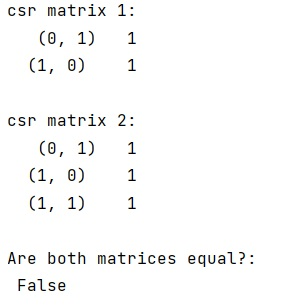# How to check if two scipy.sparse.csr_matrix() are equal?

In this tutorial, we will learn How to check if two scipy.sparse.csr_matrix() are equal in Python NumPy? By Pranit Sharma Last updated : May 05, 2023

The sparse matrix representation outputs the row-column tuple where the matrix contains non-zero values along with those values.

Suppose that we are given two scipy sparse matrices and we need to check if these matrices are equal or not.

## Check if two scipy.sparse.csr_matrix() are equal

To check if two scipy.sparse.csr_matrix() are equal, you can use a hybrid approach of numpy and scipy, use numpy.array_equal() method which takes two arrays and returns True if the input arrays are equal; False, otherwise. Convert scipy matrix to dense matrix by using .todense() method so that these matrices can become input for array_equal().

Let us understand with the help of an example,

## Python program to check if two scipy.sparse.csr_matrix() are equal

```# Import numpy
import numpy as np

# Import scipy sparse
from scipy import sparse

# Creating two scipy sparse matrix
a = sparse.csr_matrix([[0,1],[1,0]])
b = sparse.csr_matrix([[0,1],[1,1]])

# Display original matrices
print("csr matrix 1:\n",a,"\n")
print("csr matrix 2:\n",b,"\n")

# Checking both matrices
res = np.array_equal(a.todense(), b.todense())

# Display result
print("Are both matrices equal?:\n",res,"\n")
```

### Output

```csr matrix 1:
(0, 1)       1
(1, 0)        1

csr matrix 2:
(0, 1)       1
(1, 0)        1
(1, 1)        1

Are both matrices equal?:
False
```

### Output (Screenshot)